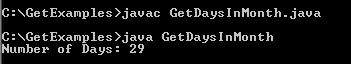Tutorials

# Java get number of Days in Month

In this section, you will learn how to obtain the number of days in the specified month.

In this section, you will learn how to obtain the number of days in the specified month.

# Java get number of Days in Month

In this section, you will learn how to obtain the number of days in the specified month.

This example sets the year as 2008, month as Calendar.FEBRUARY and the date as 1 using the method calendar.set(year, month, date) provided by the class Calendar. The Calendar class extends Object class. Calendar class provides a getInstance()  method that returns a Calendar object. Here we are going to get the number of days in the month February.

Here is the code of GetDaysInMonth.java

 ``` import java.util.Calendar;   public class GetDaysInMonth {   public static void main(String[] args)   {   Calendar calendar = Calendar.getInstance();   int year = 2008;   int month = Calendar.FEBRUARY;   int date = 1;   calendar.set(year, month, date);   int days = calendar.getActualMaximum(Calendar.DAY_OF_MONTH);   System.out.println("Number of Days: " + days);   } }```

Output will be displayed as: